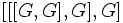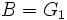# Lower central series is strongly central

This fact is an application of the following pivotal fact/result/idea: three subgroup lemma
View other applications of three subgroup lemma OR Read a survey article on applying three subgroup lemma

## Statement

The Lower central series (?) of a Nilpotent group (?) is a Strongly central series (?).

## Explanation

Intuitively, what we're saying is that the slowest way to make commutators fall is by bracketing them completely to one side. Thus, for instance, doing a bracketing like:$[[[G,G],G],G]$

is bigger than the subgroup:$[[G,G],[G,G]]$

This is closely related to the fact that the property of being a nilpotent group, which is characterized by the lower central series reaching the identity, is substantially stronger than the property of being a solvable group, which is characterized by the derived series reaching the identity.

## Facts used

1. Three subgroup lemma

## Proof

Given: A nilpotent group$G$, the lower central series of$G$ defined by$G_1 = G$,$G_m = [G,G_{m-1}]$

To prove:$[G_m, G_n] \le G_{m+n}$

Proof: We prove the result by induction on$n$ (letting$m$ vary freely; note that we need to apply the result for multiple values of$m$ for the same$n$ in the induction step).

Base case for induction: For$n = 1$, we have equality:$[G_m, G] = G_{m+1}$

Induction step: Suppose we have, for all$m$, that$[G_m,G_{n-1}] \le G_{m+n-1}$. Now, consider the three subgroups:

•$A = G_{n-1}$
•$B = G_1$
•$C = G_m$

Applying the three subgroup lemma to these yields that$[[G_{n-1}, G_1],G_m]$ is contained in the normal closure of the subgroup generated by$[[G_1,G_m],G_{n-1}]$ and$[[G_m,G_{n-1}],G_1]$.

We have:

•$[[G_1,G_m],G_{n-1}] = [G_{m+1},G_{n-1}] \le G_{m+n}$ (by induction assumption)
•$[[G_m,G_{n-1}],G_1] \le [G_{m+n-1},G_1] = G_{m+n}$ (where the first inequality is by induction assumption)

Since$G_{m+n}$ is normal, the normal closure of the subgroup generated by both is in$G_{m+n}$, hence the three subgroup lemma yields:$[[G_{n-1},G_1],G_m] \le G_{m+n} \implies [G_n,G_m] \le G_{m+n}$

which is what we require.